# Probability

Probability deals with measurements of uncertainty. A probability can be expressed as a percentage between 0% and 100%, inclusive, or as a number between 0 and 1, inclusive.

We assign probabilities to events or outcomes. For example, if we say, the probability it will rain tomorrow is 0.6, the event is it will rain tomorrow, and the assigned probability is 0.6. We can write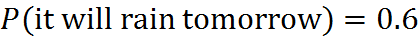If we choose A to represent the event it will rain tomorrow, the above expression is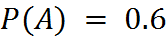In another example, a fair die will turn up 1, 2, 3, 4, 5 or 6 with equal probability each time it is tossed. Let B be the event that it turns up 1 in the next toss. We write: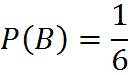## Discrete Probability

Probability comprises many concepts, but all questions about probability on the GMAT concern discrete probability. The word “discrete” here refers to situations in which all possible outcomes are discrete, or distinct and countable (rather than, say, a continuum of possibilities). For example, when rolling a six-sided die, there are 6 discrete outcomes.

One overarching principle for discrete probability questions – and, hence, for probability questions on the GMAT – is: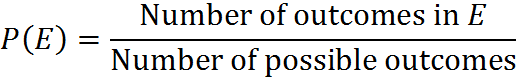In order to form this fraction and hence compute a probability of interest, you must count two categories of outcomes. One of those counts goes into the numerator and the other into the denominator. The count in the denominator is that of all possible outcomes, and the count in the numerator is that of all relevant outcomes – all the outcomes that represent the event E happening, since we want the probability of E.

This formula applies only when the outcomes are equally likely. For example, in the case of a rolling a “1” on a six-sided die, there are 6 possible outcomes and one outcome representing “1,” so again we obtain the probability 1/6.

Sometimes, people like to express this formula as the number of “desired” outcomes divided by the total number of possible outcomes. The word “desired” is imprecise, because we may or may not “desire” the outcome E, but that word may help you to remember the formula.

Notice that this formula involves counting, so one of more of the counting principles discussed in Counting Methods, near the end of this book, may be needed to calculate a probability of this type.

## Impossible and Certain Events

Two types of events are special. There are impossible events (e.g., a roll of a die will turn up 7) and events that are certain to happen (e.g., a roll of a die will turn up as one of 1, 2, 3, 4, 5 or 6). The probability of an impossible outcome is 0, while that of a certain outcome is 1. We write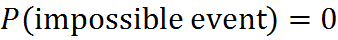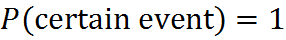The above reinforces that the range of a probability is from 0 to 1. You can never have a probability of, say, 2.5. In order words, for any event E, the probability must be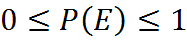## Complement of an Event

The complement of an event E is the set of one or more possible outcomes that occur when E does not occur. The complement may consist of a single possibility or a range of possibilities.

In the above case of a die, if E is the event of obtaining a particular side of the die, say 1, then the complement is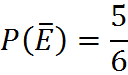The probability that the die will turn up 2, 3, 4, 5 or 6 in the next toss is 5/6. Putting a bar over a variable E means the opposite of the event E.

Note that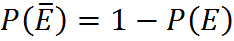for any event E.

If events are mutually exclusive, their probabilities can be added: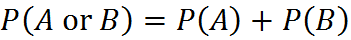Consider rolling a single die; if we want to calculate the probability for, say, rolling an odd number, we must add up the probabilities for all the “ways” in which this can happen – rolling a 1, 3, or 5. Consequently, we come to the following calculation: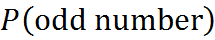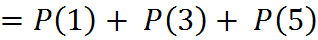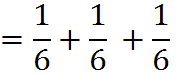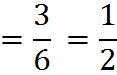Note that the addition of probabilities is often associated with the use of the word “or” – whenever we say that some event E consist of the events X, Y, or Z (being satisfied if any of the events occur) we use addition to combine their probabilities (if they are disjoint, see below).

If events are not mutually exclusive – if they can occur together – then we cannot add their probabilities in a straightforward manner. Suppose that, given a group of people, we want to know the probability that a person is female or over 30 years old. These conditions are not mutually exclusive: we may select a person who is both female and 30 years old. Since the categories overlap, if we added the probabilities simply, we would double-count some of the outcomes that give us E. If the word “double-counting” sounds familiar and reminds you of our Venn diagram discussion, you’re right on the mark: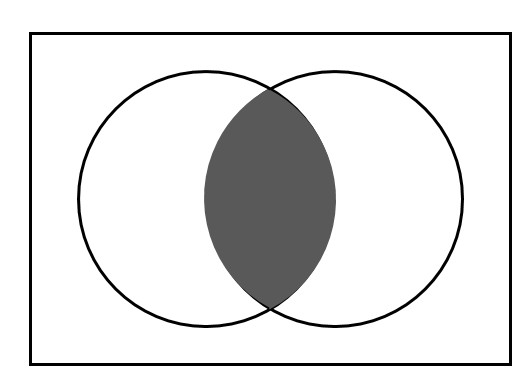Say the left circle A is the number of women, and the right circle B is the number of people over 30. The box represents the entire group T. We want to know the odds that we choose someone in one of the circles. If we add the numbers of people in the circles, we double-count the shaded area. Therefore, we must subtract it once, and the probability will be: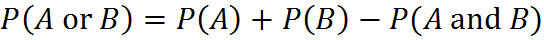This equation (which is called the Simple Inclusion-Exclusion Principle) is worth remembering. Notice that you could also rearrange it: you could use this formula to infer the odds of A and B occurring together (the rightmost term) if you knew the other three pieces. As with the formula for overlapping sets, a great way to remember this formula is to picture or draw the Venn diagram to jog your memory. And you’ll want to think of the Venn diagram when you are dealing with probabilities of events that are not mutually exclusive – which can occur together.

## Dependent Probabilities: Multiplying

Probabilities are multiplied together whenever the events concerned are dependent. We deal with a dependent probability, for example, when we calculate the overall probability of a series of independent events. For example, consider rolling a single die twice; the probability of rolling a 6 in two consecutive rolls is calculated by multiplying the probabilities for the individual rolls. Therefore, the probability of rolling a 6 twice is: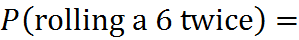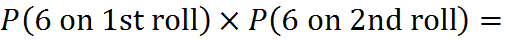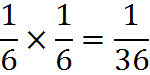The multiplication of probabilities is often associated with the use of the word “and” – whenever we say that some event E is equivalent to all of the events X, Y, and Z occurring, we use multiplication to combine their probabilities (if they are independent).

Also, it is important to recognize that the product of multiple probabilities must be less than or equal to each of the individual probabilities, since probabilities are restricted to the range 0 through 1. This agrees with our intuitive notion that relatively complex events are usually less likely to occur.

It is often necessary to use both of these operations simultaneously. Once again, consider a die being rolled twice in succession. In contrast with the previous case, we will now consider the event of rolling two numbers that add up to 3. In this case, there are clearly two steps involved, and therefore multiplication will be used, but there are also multiple ways in which the event under consideration can occur, meaning addition must be involved as well. The die could turn up 1 on the first roll and 2 on the second roll, or 2 on the first and 1 on the second. This leads to the following calculation: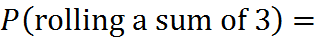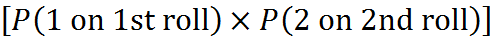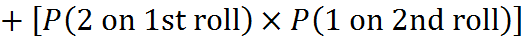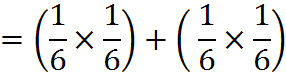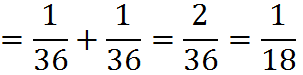This type of calculation appears fairly frequently on GMAT questions.

## Exercises

Let A represent the number that turns up in a (fair) die roll, let C represent the number that turns up in a separate (fair) die roll, and let B represent a card randomly picked out of a deck:

1. A die is rolled. What is the probability of rolling a 3 i.e. calculate P(A = 3)?

2. A die is rolled. What is the probability of rolling a 2, 3, or 5, i.e. calculate P(A = 2, 3 or 5)?

3. What is the probability of choosing a card of the suit Diamonds (in a 52-card deck)?

4. A die is rolled and a card is randomly picked from a deck of cards. What is the probability of rolling a 4 and picking the Ace of Spades?

5. Two dice are rolled together. What is the probability of getting a 1 and a 3?

6. Two dice are rolled separately. What is the probability of getting a 1 and a 3, regardless of order?

7. Calculate the probability of rolling two dice that add up to 7.

8. Let C be the number rolled on the first die and A be the number rolled on the second die. Show that the probability of C being equal to A is 1/6.

9. Let C and A be as in exercise 8. What is the probability that C is greater than A?

## Solutions

1.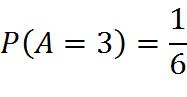2.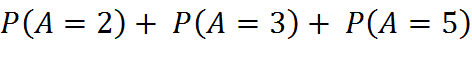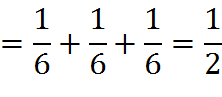3.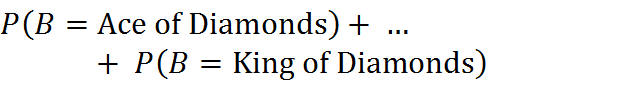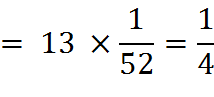4.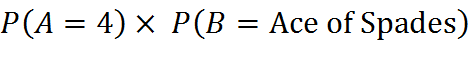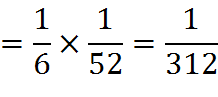5.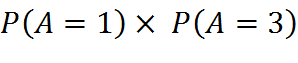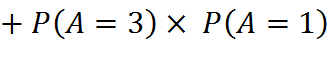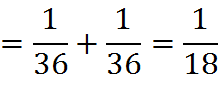6.This is the same answer as the problem above because in both cases the outcome for each individual die remains independent of the other regardless of whether or not they are thrown simultaneously. Another way of calculating the same answer is to consider that the first die can be a one or a three but the second can only be one number – the opposite of the first die, i.e. a 3 if the first die was 1, or a 1 if the first die was 3.

That gives: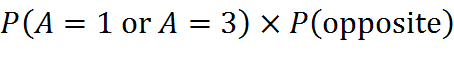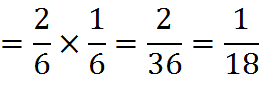7. Here are the possible combinations: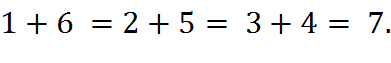The probability of getting each of the combinations is 1 in 18, as in exercise 6. There are 3 such combinations, so the probability is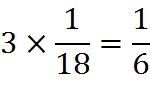8.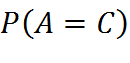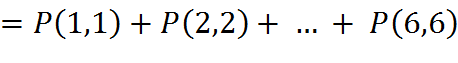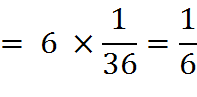9. Since both die are fair, C > A is just as likely as C < A. So we can represent both of those probabilities by the same variable, X: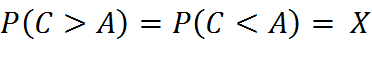and there is only one other possibility, which is that A = C. Since they covers all possibilities, the sum of probabilities must equal 1: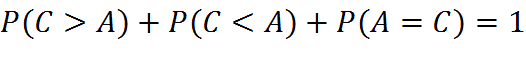And we can calculate the probability that A = C: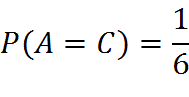Subtracting that out of the three totaling to 1, we learn that the two (equal) probabilities equal…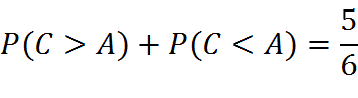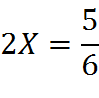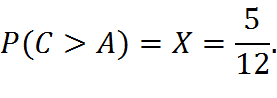## Expectation Value

Expectation values are not explicitly mentioned in the official GMAT rubric, but they can be useful to understand some GMAT questions.

An expectation value can be thought of as the long-term average of the outcome of a certain repeatable random experiment. For example, if you were to roll the die a large number of times, you would expect each of the numbers to turn up roughly an equal number of times. So the expectation is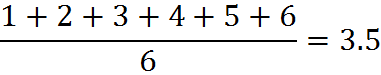We denote the expectation of D by E(D), so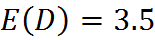If you look back at weighted averages, which we have previously discussed, you can see that expectation values are similar. An expectation value is like an overall average outcome of different possible events, weighted by their probabilities.

## Practice Questions

Identification Numbers:
http://www.gmatfree.com/identification-numbers

Probability of Pairs of Draws:
http://www.gmatfree.com/probability-of-pairs-of-draws

The Probability of a Blue Ball:
http://www.gmatfree.com/the-probability-of-a-blue-ball

Probability of a Product:
http://www.gmatfree.com/probability-of-a-product

Welcome! You are encouraged to register with the site and login (for free). When you register, you support the site and your question history is saved.